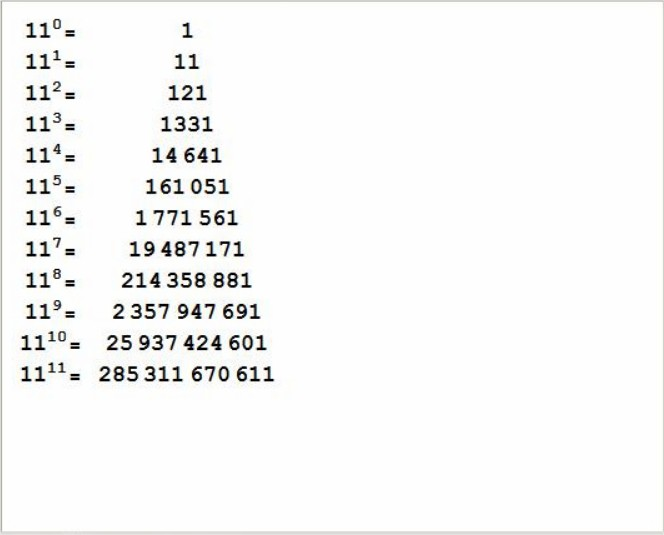# Java Program To Calculate Power Of Number | 4 Ways

Java Program To Calculate Power Of Number – In this article, we will detail in on the several ways to calculate the power of a number in Java programming.

Suitable examples and sample programs have also been added so that you can understand the whole thing very clearly. The compiler has also been added with which you can execute it yourself.

The several methods used in this specific piece are as follows:

• Using Standard Method
• Using For Loop
• Using Command Line Arguments
• Using Function
• Using Class
• Using Recursion

As we all know, the power of a number is the times a number is multiplied with itself. The power of a number, in more simpler terms, is the number of times you have to multiply the number by itself.For example, 5^3 is called as 5 to the power of 3.

Here, 5^3 = 5*5*5 = 125.

Similarly, 5^(-2) = (1/5)^2 = 1/25.

The power of 0 will always give the result as 1, no matter what the number is, unless if it is undefined or infinite.

As given in the photo above, the powers of the number 11 from 0 to 11 are mentioned there. It is clearly seen that 11 is multiplied by the result once after the other as the power increases.

Thus, the manifold ways to calculate the power of a number in Java programming are as follows:

Output:

Output:

Output:
Output:
Output:
Output:
x

## X Star Pattern Java Program – Patterns

Java program to print X star pattern program – We have written the below print/draw ...